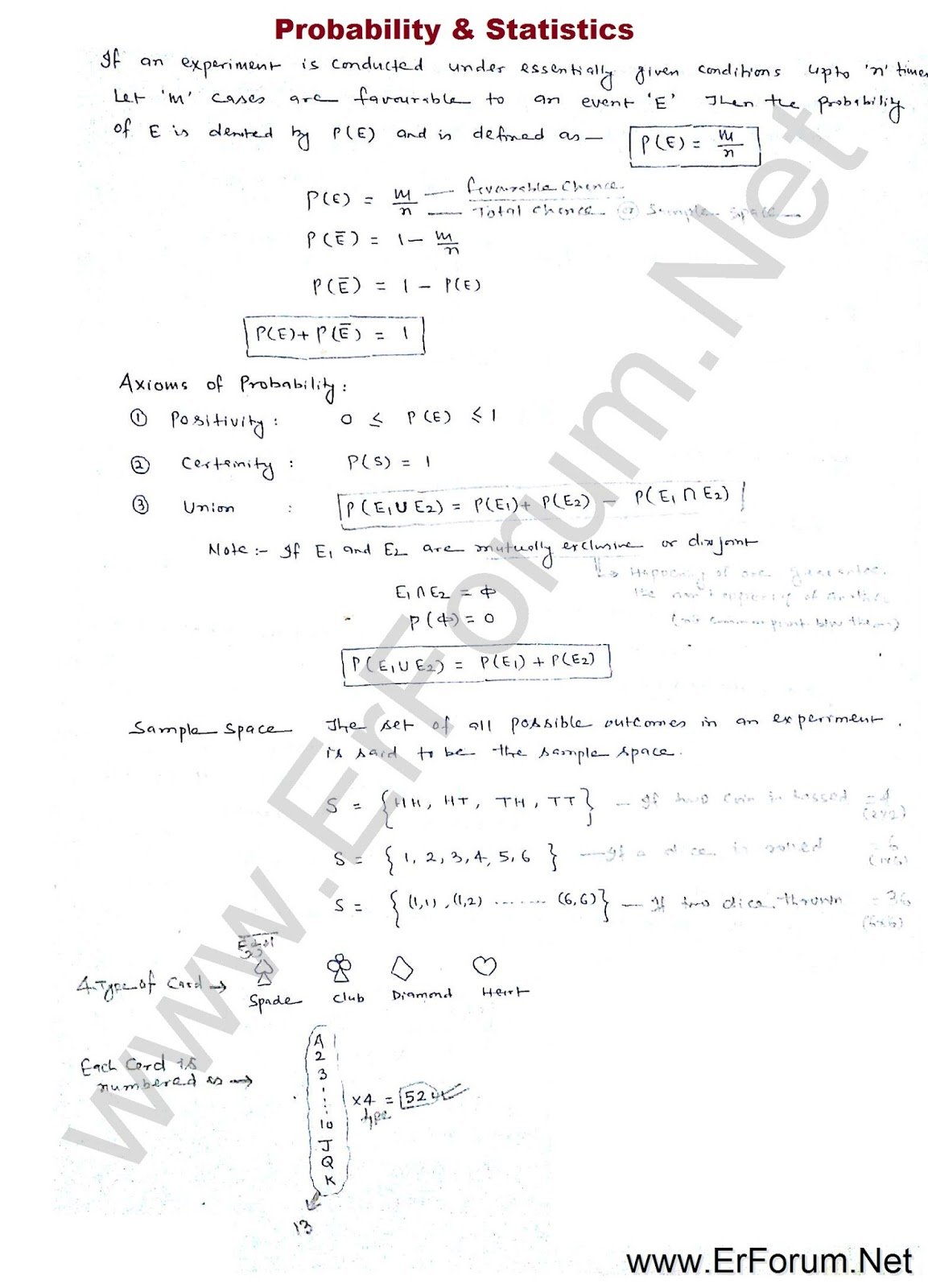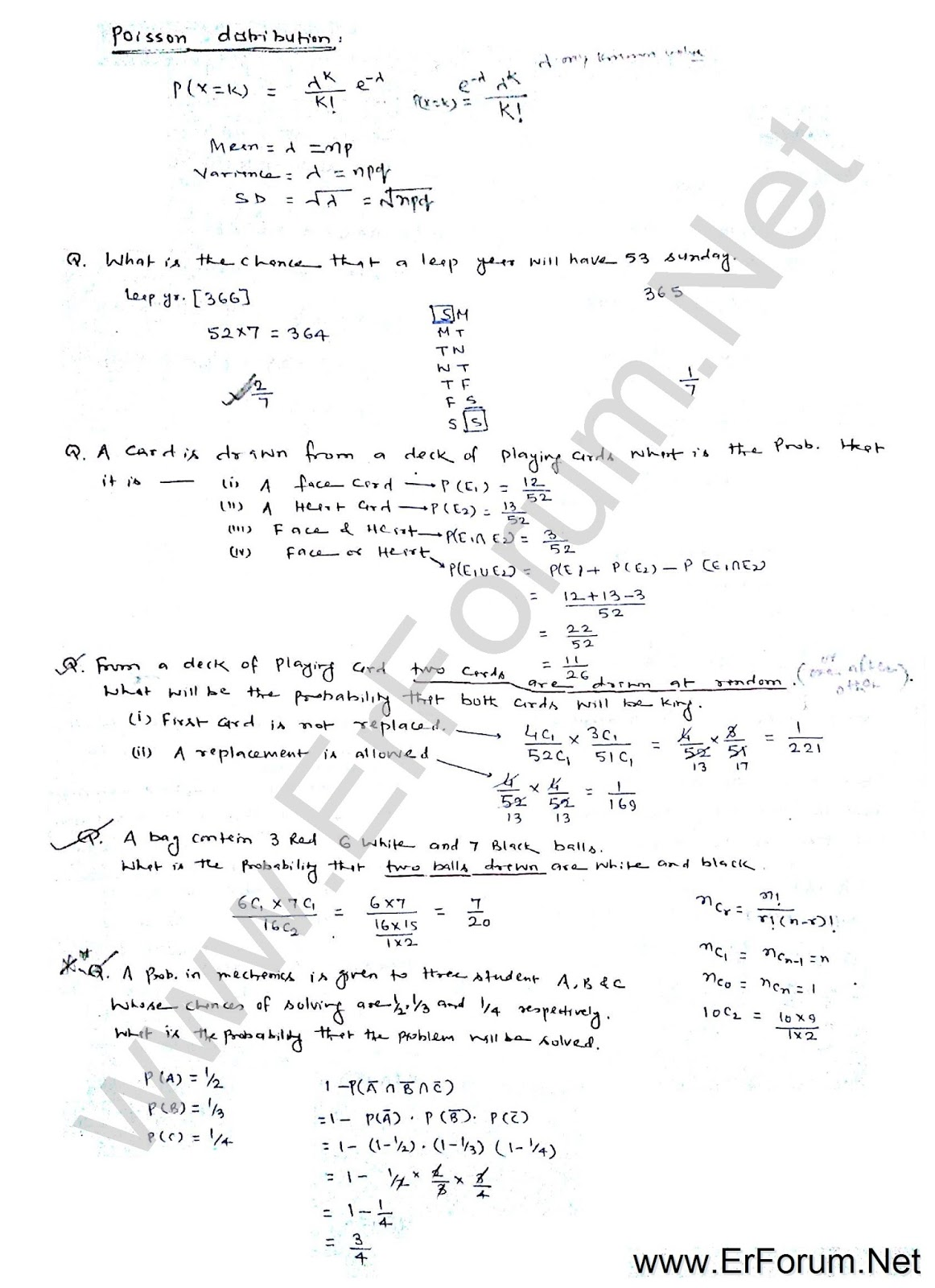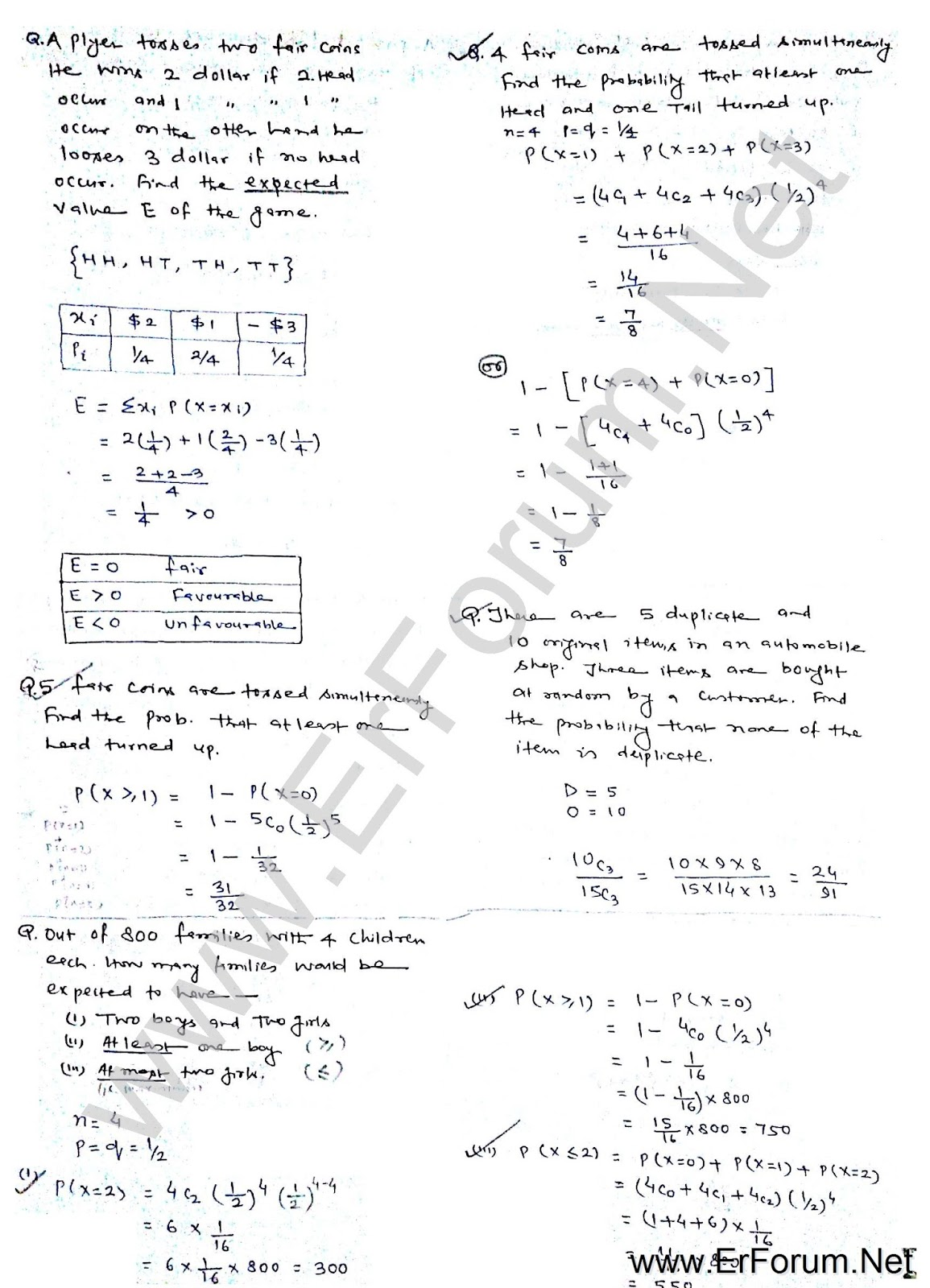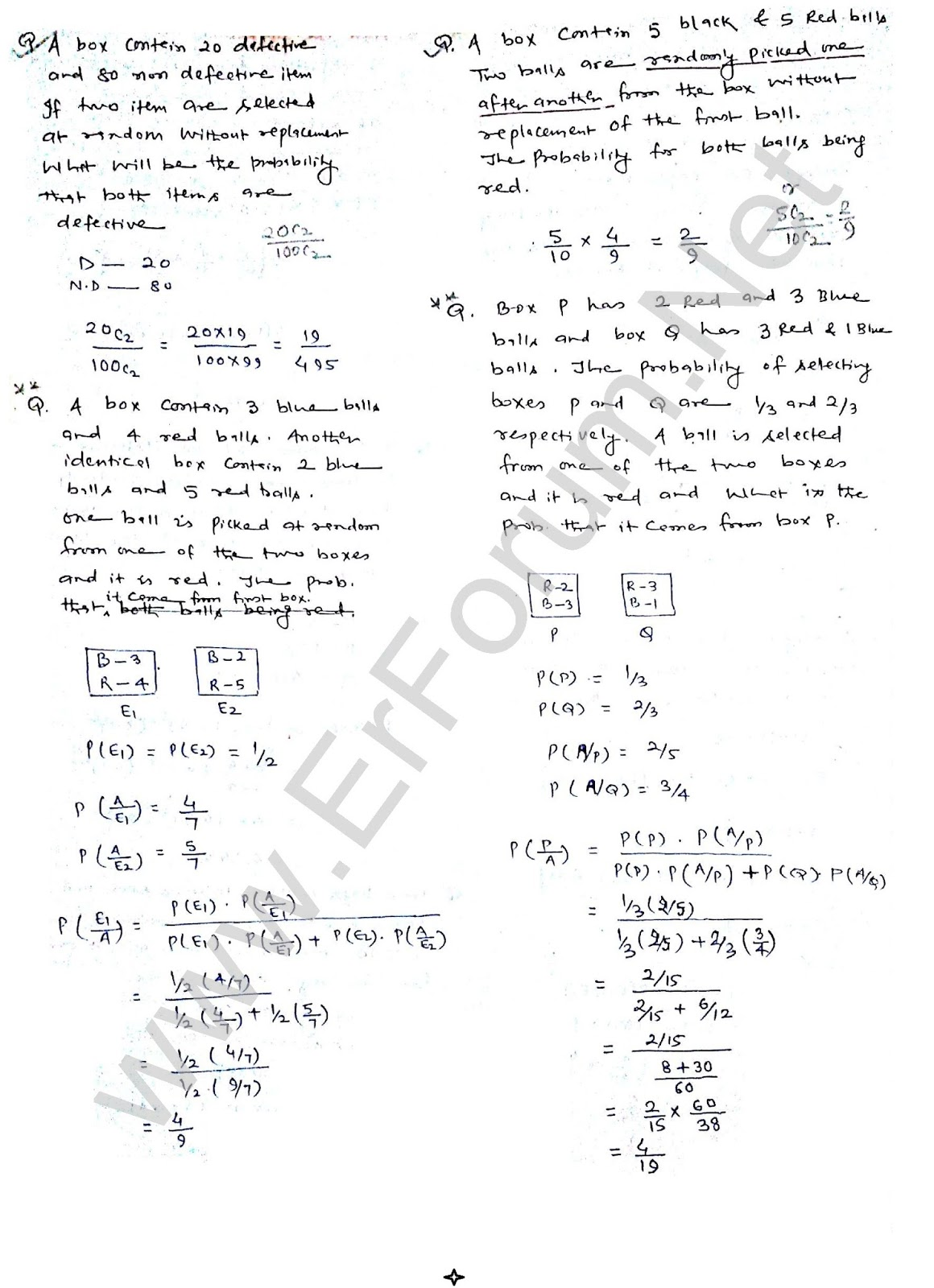3/recent/ticker-posts

# Probability & Statistics for GATE Exams

### Probability:

Probability and statistics is one of the most scoring topics of engineering math for GATE Exam. Each year there is confirmed question from this topic. You should read this topic very well to score. Here in this post, we are sharing all concepts of Probability and statistics in the form of handwritten notes. You can download these notes from below link.Probability & Statistics Notes-1
Probability & Statistics Notes-2
Probability & Statistics Notes-3

If you want to read these concepts with tricks read below notes:For Complete Engineering Math of GATE Exam Visit this Link
Your Like and Share motivate us to share more such notes. So, please Like & Share it.